# Texas Go Math Grade 3 Lesson 18.4 Answer Key Fractions in Measurements

Refer to our Texas Go Math Grade 3 Answer Key Pdf to score good marks in the exams. Test yourself by practicing the problems from Texas Go Math Grade 3 Lesson 18.4 Answer Key Fractions in Measurements.

## Texas Go Math Grade 3 Lesson 18.4 Answer Key Fractions in Measurements

Unlock the Problem

Steve ran $$\frac{3}{4}$$ mile and Jenna ran $$\frac{4}{4}$$ of a mile. Did Steve and Jenna run the same distance?

Math Idea
If two numbers are located at the same point on a number line, then they are equal and represent the same distance from zero.

Locate $$\frac{3}{4}$$ and $$\frac{4}{4}$$ on a number line.

• Label the number line.
• Draw a point at $$\frac{3}{4}$$ and $$\frac{4}{4}$$.Since the distance ________ and _______ are not at the same point, they are not the same distance from zero.
__________, Steve and Jenna did not run the same distance.
$$\frac{3}{4}$$ and $$\frac{4}{4}$$.

Explanation: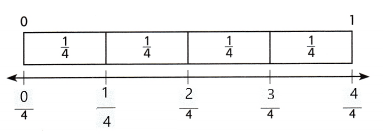The distance $$\frac{3}{4}$$ and $$\frac{4}{4}$$ is not at the same point, they are not the same distance from zero.
Hence, Steve and Jenna did not run the same distance.

Try This! Complete the number line. Locate and draw points at $$\frac{1}{2}$$ and $$\frac{2}{2}$$.Think: Do the distances end at the same point?
A. Are $$\frac{1}{2}$$ and 1 the same distance from zero? Explain.
No, they are not at the same point

Explanation:The distance $$\frac{1}{2}$$ and $$\frac{1}{2}$$ is not at the same point, they are not the same distance from zero

B. Are $$\frac{2}{2}$$ and 1 the same distance from zero? Explain.
Yes, they are at the same point.

Explanation:
The given fractions $$\frac{2}{2}$$ and 1 the same distance from zero which is mentioned in the number line.

Share and Show

Beth walked $$\frac{5}{8}$$ mile. Jodi walked $$\frac{7}{8}$$ mile. Austin watched the girls walk. Did Beth, Jodi, and Austin walk the same distance?

Complete the number line. Locate and draw points that Beth, Jodi, and Austin walked.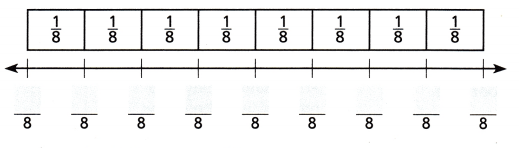Think: Do the distances end at the same point?

Question 1.
How far did Austin walk?
Austin watched the girls watch. Therefore he is at the same point as in the beginning.

Explanation: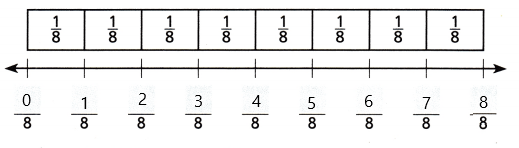Question 2.
Who walked the farthest distance? Explain.
Jodi

Explanation:
Jodi walked the farthest distance.

Use the number line to find which fraction is farther from zero or if the fractions are the same distance from zero.Question 3.
$$\frac{1}{8}$$ and $$\frac{3}{4}$$
$$\frac{3}{4}$$

Explanation:
The fraction $$\frac{3}{4}$$ is farther from zero in the number line.

Question 4.
$$\frac{1}{4}$$ and $$\frac{2}{8}$$
Both fractions have same distance in the number line.

Explanation:
The fraction $$\frac{1}{4}$$ and $$\frac{2}{8}$$ are in the same distance from zero.

Question 5.
$$\frac{2}{4}$$ and $$\frac{4}{8}$$
Both fractions have same distance in the number line.

Explanation:
The fraction $$\frac{2}{4}$$ and $$\frac{4}{8}$$ are in the same distance from zero.

Question 6.
$$\frac{5}{8}$$ and $$\frac{7}{8}$$

Problem Solving

H.O.T. What’s the Error?

Question 7.
Andrea and Elaina were making material bags. Andrea bought $$\frac{4}{8}$$ of a yard of material and Elaina bought $$\frac{1}{2}$$ yard of material. Elaina said she bought more material than Andrea did.Analyze Look at how Elaina solved the problem. Find her error.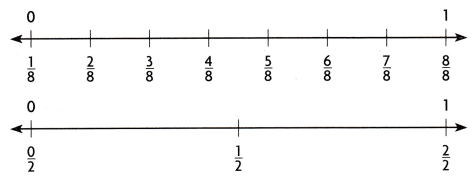Use Math Language Describe Elaina’s error.
No, Elaina and Andrea bought the same amount of material.

Explanation:
Andrea bought $$\frac{4}{8}$$ of material and Elaina bought $$\frac{1}{2}$$ of material. Therefore by comparing both we can say that both bought the same amount of material.

Question 8.
H.O.T. Multi-Step Jeff rode his bike around a bike trail that was $$\frac{1}{4}$$ mile long. He rode around the trail 4 times. Dexter rode around the trail 2 times. Complete the number line to show the distances that Jeff and Dexter rode.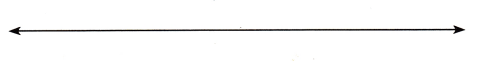Use Tools Did Dexter ride the same distance as Jeff? Explain how you know.
No. Dexter rides the same distance as Jeff.

Explanation:
Jeff rode his bike at $$\frac{1}{4}$$ mile long. He rode 4 trails. That means jeff rode at a fraction of $$\frac{4}{4}$$.
Dexter rode around 2 trail times. That means Dexter rode $$\frac{2}{4}$$.

Fill in the bubble for the correct answer choice.

Use a number line to help solve the problem.

Question 9.
A sloth moves $$\frac{1}{4}$$ meter each second on the ground. How far does the sloth move in 4 seconds?(A) 1 meter
(B) $$\frac{1}{2}$$ meter
(C) 2 meters
(D) $$\frac{1}{4}$$ meter
1 meter

Explanation:
The sloth moves 11 meter in 4 seconds.

Question 10.
On weekends, Salma’s family hikes at the state park. They hike $$\frac{1}{4}$$ of a mile every 30 minutes. How much of a mile do they hike in 90 minutes?
(A) $$\frac{1}{2}$$ mile
(B) $$\frac{1}{4}$$ mile
(C) $$\frac{3}{4}$$mile
(D) 1 mile
$$\frac{3}{4}$$mile

Explanation:
They hike $$\frac{3}{4}$$mile in 90 minutes.

Question 11.
Multi-Step *(A) $$\frac{2}{8}$$
(B) $$\frac{5}{8}$$
(C) $$\frac{8}{8}$$
(D) $$\frac{3}{8}$$
$$\frac{8}{8}$$

Texas Test Prep

Question 12.
Which fraction is the farthest distance from zero?
(A) $$\frac{1}{4}$$
(B) $$\frac{1}{2}$$
(C) $$\frac{2}{4}$$
(D) $$\frac{6}{8}$$
$$\frac{6}{8}$$

Explanation:
The fraction is the farthest distance from zero is $$\frac{6}{8}$$.

### Texas Go Math Grade 3 Lesson 18.4 Homework and Practice Answer Key

Use the number line to find which fraction is farthest from zero or the same distance.Question 1.
$$\frac{2}{8}$$ and $$\frac{1}{4}$$
The given fraction has same distance.

Explanation:
$$\frac{2}{8}$$ and $$\frac{1}{4}$$ has a same distance.

Question 2.
$$\frac{3}{8}$$ and $$\frac{2}{4}$$
$$\frac{2}{4}$$ is farthest from zero.

Explanation:
The given fraction $$\frac{2}{4}$$ is farthest from zero.

Question 3.
$$\frac{3}{4}$$ and $$\frac{5}{8}$$
$$\frac{5}{8}$$ is farthest from zero.

Explanation:
The given fraction $$\frac{5}{8}$$ is farthest from zero.

Question 4.
$$\frac{7}{8}$$ and $$\frac{3}{4}$$
$$\frac{7}{8}$$

Explanation:
The given fraction $$\frac{7}{8}$$ is farthest from zero.

Question 5.
$$\frac{5}{8}$$ and $$\frac{1}{4}$$
$$\frac{5}{8}$$

Explanation:
The given fraction $$\frac{5}{8}$$ is farthest from zero.

Question 6.
$$\frac{3}{4}$$ and $$\frac{6}{8}$$
The both given fractions has same distance.

Explanation:
The given fractions $$\frac{3}{4}$$ and $$\frac{6}{8}$$ has same distance which are in the number line.

Problem Solving

Question 7.
Harley and Noreen were making kites. Harley has $$\frac{3}{4}$$ of a yard of yellow kite string. Noreen has $$\frac{6}{8}$$ of a yard of green kite string. She says that she has more kite string than Harley. How can Harley draw a number line to show that Noreen is incorrect? Write a sentence to explain how you solved the problem.No, the statement is incorrect.

Explanation:
They both have the same amount of string. As Harley has $$\frac{3}{4}$$ of a yard of yellow string. Noreen has $$\frac{6}{8}$$ of a yard of the green kite. Hence they both have the same amount of string.

Question 8.
Harley gets another $$\frac{1}{8}$$ yard of kite string. How much string does he have now?
$$\frac{7}{8}$$

Explanation:
As Harley has $$\frac{3}{4}$$ and another $$\frac{1}{8}$$ yard. Therefore the string he has now is $$\frac{3}{4}$$+$$\frac{1}{8}$$ = $$\frac{3}{4}$$.

Lesson Check

Question 9.
A flock of geese fly $$\frac{1}{4}$$ mile every 20 minutes. How far do the geese travel in 1 hour?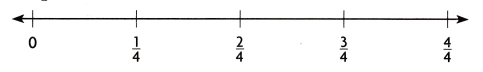(A) $$\frac{3}{4}$$ mile
(B) $$\frac{1}{4}$$ mile
(C) $$\frac{2}{4}$$ mile
(D) $$\frac{4}{4}$$ mile
$$\frac{3}{4}$$ mile

Explanation:
$$\frac{1}{4}$$ in 20 minutes
The geese travel in 1 hour is $$\frac{3}{4}$$ mile.

Question 10.
Adam uses an inch ruler to measure and cut the ribbon for an art project. Which statement best describes the pieces that are $$\frac{3}{4}$$ inch and $$\frac{7}{8}$$ inch?
(A) the $$\frac{3}{4}$$ inch piece is farther from zero on the ruler
(B) the pieces are the same distance from zero on the ruler
(C) the $$\frac{7}{8}$$ inch piece is farther from zero on the ruler
(D) the $$\frac{7}{8}$$ inch piece is closer to zero on the ruler
the $$\frac{7}{8}$$ inch piece is farther from zero on the ruler

Question 11.
Multi-Step An ant travels $$\frac{1}{8}$$ yard to an anthill every second. How far does the ant go in 6 seconds?(A) $$\frac{3}{4}$$ yard
(B) $$\frac{1}{4}$$ yard
(C) $$\frac{2}{4}$$ yard
(D) $$\frac{7}{8}$$ yard
$$\frac{3}{4}$$ yard

Explanation:
For 1 second  an ant traveled $$\frac{1}{8}$$
For 6 second the ant travelled for $$\frac{3}{4}$$ yard

Question 12.
Multi-Step Edwin runs $$\frac{1}{8}$$ mile around a track in 2 minutes. He runs for 4 minutes. Then he walks for another $$\frac{3}{8}$$ mile. How far does Edwin go around the track?(A) $$\frac{3}{8}$$ mile
(B) $$\frac{4}{8}$$ mile
(C) $$\frac{2}{8}$$ mile
(D) $$\frac{5}{8}$$ mile